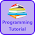# Programming Videos

## Search

### C, C++ Program to Find Second Largest Element in an Unsorted Array

Write a C, C++ program to find second largest element in an array. Given an unsorted array, we have to write a code to find second largest element in an array.

There is multiple approach to find the second element number in an array. In my previous tutorial, I have explained how to find second largest number using sorting.

We can solve this problem without using sorting as well in O(n) time complexity.

## C++ Program to Find Second Largest Element in an Unsorted Array

```#include <iostream>

using namespace std;

int main() {

int n, i;

cout << "Enter number of elements in an array \n";
cin  >> n;

int arr[n];

cout << "Enter values in an array \n";

//Input array elements
for (i = 0; i < n; i++) {
cin >> arr[i];
}

//Assign min value to max and second_max
int max         = INT_MIN;
int second_max  = INT_MIN;

for (i = 0; i < n; i++) {

if(arr[i] > max) {
second_max = max;
max        = arr[i];
}

if(arr[i] < max && arr[i] > second_max) {
second_max = arr[i];
}
}

cout << "Second highest number in an unsorted
array is " << second_max;

return 0;
}

```

### C Program to Find Second Largest Element in an Array Without Using Sorting

```#include <stdio.h>

main()
{

int arr[] = {4,5,2,1,7,8};

int highest=0, i;
int secondhighest = 0;

int n = sizeof(arr)/sizeof(arr);

/* Traverse an array. */

for(i=0;i<n;i++){

/* If value of arr[index] is greater than highest value. */

if(arr[i]>highest){

secondhighest = highest;
highest = arr[i];

}else if(arr[i]>secondhighest && arr[i] !=highest){

secondhighest = arr[i];

}
}

printf("Second highest is %d",secondhighest);

}
```

1.2.